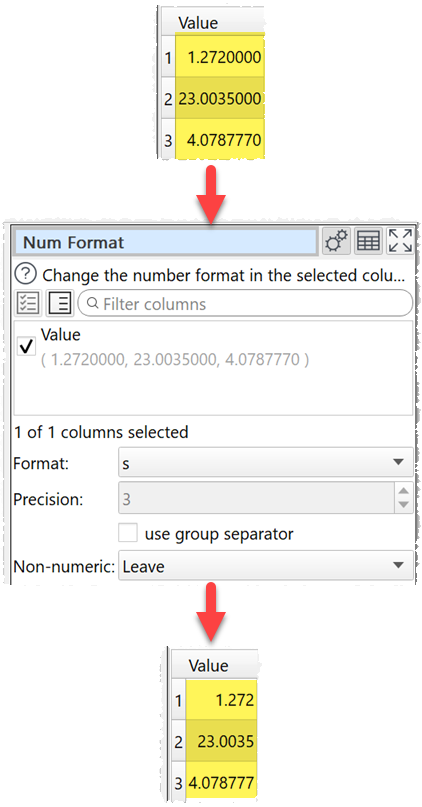﻿ Reference > Transforms > Num Format

# Num Format

## Description

Change the number format in one or more columns.

## Examples

Set numeric values to 2 decimal places:Set numeric to shortest accurate number:One.

## Options

Check the column(s) you wish to transform.

Set Format to the new number format (see below).

For the e, E, and f formats, Precision represents the number of digits after the decimal point. For the g and G formats, Precision represents the maximum number of significant digits (trailing zeros are omitted). For the s format Precision is ignored. The following number formats are supported:

Format

Meaning

e

Format as [-]9.9e[+|-]999.

E.g. 1234567.89 is shown as 1.235e+06.

E

Format as [-]9.9E[+|-]999.

E.g. 1234567.89 is shown as 1.235E+06.

f

Format as [-]9.9.

E.g. 1234567.89 is shown as 1234567.89.

g

Use e or f format, whichever is the most concise.

G

Use E or f format, whichever is the most concise.

s

The shortest accurate representation for the given number without exponents.

E.g. 1234567.00 is shown as 1234567.

Check use group separators to include the group separators for your locale. E.g. to turn 1234567 to 1,234,567 for a UK or US locale.

Set Non-numeric according to what you want to do with non-numeric values in transformed columns. NaN means Not a Number.

## Notes

Numbers should be base 10 (decimal).

The locale is used to decide how the number is represented (e.g. group and decimal separators).

Non-numerical values are ignored.

You can also use Extract and Pad to change the number of characters.

Warnings are shown in the Warnings tab for non-numeric values.